SCERT AP 7th Class Maths Solutions Pdf Chapter 7 Ratio and Proportion Ex 7.5 Textbook Exercise Questions and Answers.

## AP State Syllabus 7th Class Maths Solutions 7th Lesson Ratio and Proportion Ex 7.5

Question 1.
Rekha bought a wrist watch for ₹ 2250 and sold it for ₹ 1890. Then find her loss or gain percentage?Given cost price of wrist watch = ₹ 2250
Selling price of wrist watch = ₹ 1890
C.P > S.P, then Rekha got loss.
Loss = C.P – S.P = 2250 – 1890 = ₹ 360

We know loss percent = $$\frac{\text { Loss }}{\text { C.P }}$$ × 100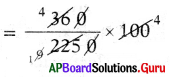∴ Loss percentage 16%.

Question 2.
A shopkeeper buys a toy for ₹ 250 and sells it for ₹ 300. Find his gain or loss percentage?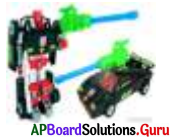Given cost price of a toy = ₹ 250
Selling price of a toy = ₹ 300
C.P < S.P, then Shopkeeper got profit.
Profit = S.P – C.P
= 300 – 250 = ₹ 50

We know profit percent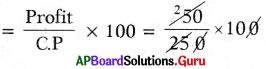∴ Profit percentage = 20%Question 3.
The cost price of a chair ₹ 480. 1f he sold at a profit of 10%. what would be selling price of it’?
Method: 1
Given cost price of a chair = ₹ 480
Profit percent = 10%
Profit = 10% of ₹ 480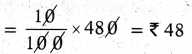Selling price = C.P + Profit
= ₹ 480 + ₹ 48
∴ Selling price = ₹ 528

Method: 2
C.P = 100% = ₹ 480
Selling it 10% gain.
= S.P = 100% + 10% =?
z 100% = ₹ 480
110% =?
S.P = $$\frac{110}{100}$$ × 480= ₹ 528
∴ Selling price = ₹ 528

Question 4.
If Sharma purchased a car for ₹ 350000. After two years he sold at a loss of 12%. Find its selling price’?
Given cost price of a car = ₹ 350000
Loss = 12 %
Loss 12% of ₹ 350000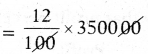Loss = ₹ 42000
Selling price = CP – Loss
= 350000 – 42000
∴ Selling price of a car = ₹ 3,08,000

Question 5.
A shopkeeper buys wooden tables each at ₹ 2800 and expends ₹ 400 on each table for painting. If he sells it at a cost of ₹ 4000. Find his profit percentage?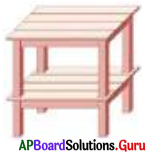Given cost price of wooden table = ₹ 2800
Amount spent on painting = ₹ 400
Effective cost price of wooden table
= ₹ 2800 + ₹ 400 = ₹ 3200

Selling price wooden table = 4000
C.P < SP, then shopkeeper got profit.
Profit = S.P – C.P
= ₹ 4000 – ₹ 3200 = ₹ 800

We know profit percentage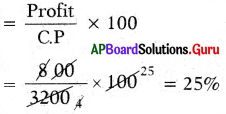∴ Profit percentage = 25%Question 6.
In a garment shop a saree is sold at a cost of ₹ 1800 after a profit of ₹ 600. Find the profit percentage and cost price?
Given selling price of a saree = ₹ 1800
Profit = ₹ 600
Cost price of a saree = S.P – Profit
= 1800 – 600
= ₹ 1200.
We know that profit percentage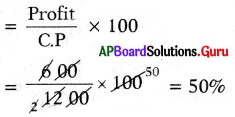∴ Profit percentage = 50%

Question 7.
After incurring a loss of ₹ 258, Jean pant was sold at ₹ 1750. Then find the cost price and loss percentage?
Given selling price of Jean pant = ₹ 1750
Loss = ₹ 258
Cost price of Jean pant = S.P + Loss
= 1750 + 258
= ₹ 2008
Loss percent = $$\frac{\text { Loss }}{\text { C.P }}$$ × 100
⇒ $$\frac{258}{2008}$$ × 100
∴ Loss percentage = 12.85%

Question 8.
The cost price of 10 articles is equal to the selling price of 9 articles. Find the profit percentage?
Method 1:
Let Cost price of 10 articles be ₹ x.
Cost price of 10 articles = 10 x
Selling price of 9 articles = 10 x
Cost price of 9 articles 9x
Profit = S.P — C.P = 10x – 9x
= ₹ x
Profit percentage = $$\frac{\text { Profit }}{\text { C.P }}$$ × 100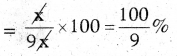∴ Profit percentage = $$\frac{100}{9}$$% (or)
11.11% (or) 11$$\frac{1}{9}$$%

Method 2:
By selling 9 articles, there is a gain of 1 article.
∴ Profit Percentage = $$\frac{1}{9}$$ × 100
= 11$$\frac{1}{9}$$%Question 9.
By selling a book for ₹ 258, a bookseller gains 20%. For how much should he sell it to gain 30%?
Method 1:
Given selling priceof a book = ₹ 258
Gain = 20%
Cost price of book = ₹ x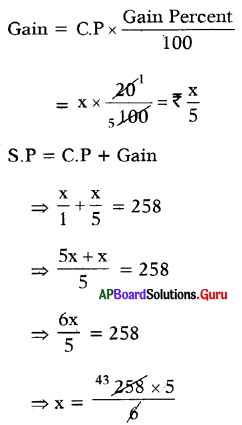∴ Cost price of a book = ₹ 215

If profit percent = 30%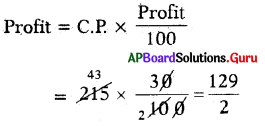Profit = ₹ 64.50
∴ S.P = C.P + Profit
= 215 + 64.50 = 279.50
To get 30% profit he has to sold at ₹ 279.50.

Method 2:
Let the C.P of the book = 100%
Selling at a gain of 20% means (100 + 20)% = ₹ 258
120% = ₹ 258
Selling at a gain of 30% means (100+30)%=?
If 120% = ₹ 258
then 130% =?
∴ SP = $$\frac{130}{120}$$ × 258 = ₹ 279.5